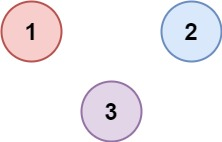0547. 省份数量

# 0547. 省份数量#

• 标签：深度优先搜索、广度优先搜索、并查集、图
• 难度：中等

## 题目大意 #

「省份」是由一组直接或间接链接的城市组成，组内不含有其他没有相连的城市。

• $1 \le n \le 200$。
• $n == isConnected.length$。
• $n == isConnected[i].length$。
• $isConnected[i][j]$ 为 $1$ 或 $0$。
• $isConnected[i][i] == 1$。
• $isConnected[i][j] == isConnected[j][i]$。

• 示例 1：1 2  输入：isConnected = [[1,1,0],[1,1,0],[0,0,1]] 输出：2 
• 示例 2：1 2  输入：isConnected = [[1,0,0],[0,1,0],[0,0,1]] 输出：3 

## 解题思路 #

### 思路 1：并查集 #

1. 遍历矩阵 isConnected。如果 isConnected[i][j] == 1，将 i 节点和 j 节点相连。
2. 然后判断每个城市节点的根节点，然后统计不重复的根节点有多少个，即为「省份」的数量。

### 思路 1：代码 #

  1 2 3 4 5 6 7 8 9 10 11 12 13 14 15 16 17 18 19 20 21 22 23 24 25 26 27 28 29 30 31 32 33 34  class UnionFind: def __init__(self, n): # 初始化 self.fa = [i for i in range(n)] # 每个元素的集合编号初始化为数组 fa 的下标索引 def find(self, x): # 查找元素根节点的集合编号内部实现方法 while self.fa[x] != x: # 递归查找元素的父节点，直到根节点 self.fa[x] = self.fa[self.fa[x]] # 隔代压缩优化 x = self.fa[x] return x # 返回元素根节点的集合编号 def union(self, x, y): # 合并操作：令其中一个集合的树根节点指向另一个集合的树根节点 root_x = self.find(x) root_y = self.find(y) if root_x == root_y: # x 和 y 的根节点集合编号相同，说明 x 和 y 已经同属于一个集合 return False self.fa[root_x] = root_y # x 的根节点连接到 y 的根节点上，成为 y 的根节点的子节点 return True def is_connected(self, x, y): # 查询操作：判断 x 和 y 是否同属于一个集合 return self.find(x) == self.find(y) class Solution: def findCircleNum(self, isConnected: List[List[int]]) -> int: size = len(isConnected) union_find = UnionFind(size) for i in range(size): for j in range(i + 1, size): if isConnected[i][j] == 1: union_find.union(i, j) res = set() for i in range(size): res.add(union_find.find(i)) return len(res) 

### 思路 1：复杂度分析 #

• 时间复杂度：$O（n^2 \times \alpha(n)）$。其中 $n$ 是城市的数量，$\alpha$ 是反 Ackerman 函数。
• 空间复杂度：$O(n)$。